# The Mathematics of Sequence Alignment

1. Alignments as Sequences of Deletions, Insertions, and Substitutions

Mathematically, the pairwise DNA-sequence alignment problem begins by providing two sequences S1 and S2 composed from the four characters A, C, G, or T. The following sequences present an example:

S1: AGTGTTCCAG

S2: AATCGTTACAG

An alignment of two sequences is a record of “edits” (or lack thereof) in the bases in S1 that leads to the sequence S2. Allowable edit operations are:

Deletion (D): delete a base

Insertion (I): insert a new base

Substitution (S): replace a base with another base

Notice that a substitution may not represent an actual “edit” as it does not rule out that a base may be “replaced” by exactly the same base. Biologically, the latter would correspond to a match. A substitution reflecting an actual change of the base would correspond to a mutation.

Example 1. Below are some possible alignments of the sequences S1 and S2. Modified strings M1 and M2 represent insertions and deletions by placing a hyphen (-) into S1 for an insertion, and into S2 for a deletion. We will refer to M1 and M2 as the alignment strings of an alignment. We can also represent an alignment as a list of edit operations L. The sequence L represents the transformation of S1 into S2 by enacting the edits listed in L on S1 from left to right. L is called the edit string of the alignment.

1. M1:    A-GTGTTCCAG
M2:    AATCGTTACAG
L:     SISSSSSSSSS                   (1 insertion, 9 substitutions, 0 deletions)

2.     M1:    -AGT-GTTCCAG
M2:    AA-TCGTTACAG
L:     ISDSISSSSSSS
(2 insertions, 8 substitutions, 1 deletion)

3.     M1:    ---------AGTGTTCCAG
M2:    AATCGTTACAG--------
L:     IIIIIIIIISSDDDDDDDD
(9 insertions, 2 substitutions, 8 deletions)

The curious reader may wonder why the same letter (S) is used to indicate both an exact match and a mismatch substitution. The distinction between an exact match and a mismatch substitution is indeed important, and the alignment tool BLAST represents this distinction by placing vertical lines between the exact matches as in the example below.

```        A-GTGTTCCAG
|   ||| |||
AATCGTTACAG
```
The advntage of a coding scheme that uses S for both matches and mismatches is that it is easy to check by simple counting whether a given edit string (L) is a valid edit string. Consider the following edit string
```IISDSSDSI
```
Each S in the edit string adds one character to each sequence, each I adds a letter only to the second sequence, and each D adds a ltter only to the first sequence. So the first sequence must have 4 + 2 = 6 letters, and the seond must have 4 + 3 = 7 letters.

This is the only condition placed on an edit string. In other words, if  #I, #D, and #S denote respectively the number of insertions, deletions, and substitutions in the sequence L, then

 A sequence L represents a valid edit string for S1 and S2 if and only if #I + #S = n = length of S2, and #D + #S = m = length of S1.

Exercise 1. Are all three alignments in Example 1 of equal biological importance? Explain why or why not.

Exercise 2. Verify that the conditions  #I + #S = 10 and #D + #S = 11 are satisfied for all of the alignments presented in Example 1.

Exercise 3. Let S1 and S2 be the same sequences as above. Which of the L's below represent a valid alignment of S1 and S2? Explain why or why not. For each valid edit string give the alignment strings M1 and M2 as was done in Example 1.

a)     L: SSSISSSSSSS

b)     L: ISSSSSSSSSS

c)      L: SSSDSSSSSSII

d)     L: SSIDSSSIDISS

2. Alignments as Paths on a Graph

Once an alignment is understood as a sequence L formed of the characters I, D, and S, a more visual way to represent that alignment is to view it as a path on the alignment graph of the sequences of S1 and S2.  If S1 has length m and S2 has length n, the alignment graph is a given as triangular lattice of height m and width n such as that in Figure 1. The DNA bases in S1 label the rows of the lattice while the DNA bases in S2 label the columns. The alignment graph of our example sequences S1 and S2 is presented in Figure 1A.Panel A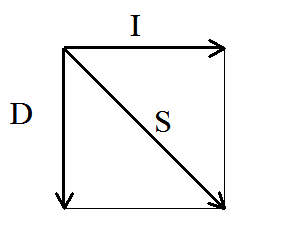Panel B Figure 1. An Alignment Graph. Panel A: The alignment graph of the sequences S1 and S2. In this case m = 10 and n = 11. Panel B: Possible directions on the graph from a vertex to an adjacent vertex.

The alignment graph is a directed graph. In directed graphs, access from one vertex to an adjacent vertex via a connecting edge is allowed only in the specified direction. For the alignment graphs we just described, the possible directions are: one horizontal step from left to right; one vertical step from top to bottom, and one diagonal step from the upper left to the lower right corner. These possibilities are depicted in Figure 1B.

A path between two vertices on the alignment graph is a walk along the edges of the graph in the permissible directions that connects these two vertices. In what follows we will only be interested in paths on the alignment graph from the upper left to the lower right vertices of the graph. In Figure 1A those vertices are marked by the symbol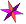. Many such paths exist, of course. Figure 2 depicts three paths that connect these vertices.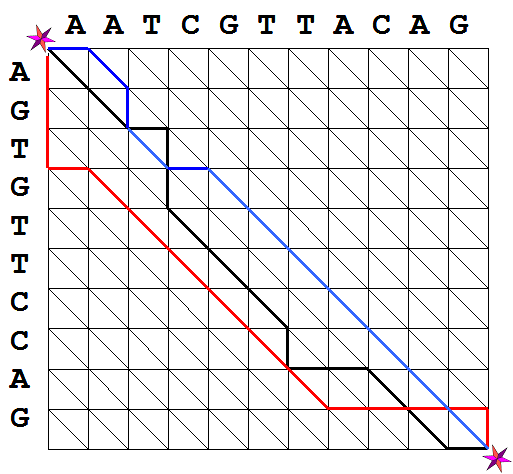Figure 2. Possible paths in the alignment graph. The blue path corresponds to the alignment presented in Exercise 1b).

Consider now Figure 1B again. If for any path on the alignment graph, a record is made for the directions followed on each step, using the labels D, I, and S, as depicted in the figure. Each path on the alignment graph represents a sequence formed from these three characters. The black path in Figure 2 then corresponds to the edit sequence
L: SSIDDSSSDIISSI and the red path corresponds to L: DDDISSSSSSIIIID. Notice that for these paths #I + #S = 10 and #D + #S = 11. The opposite is also true: any sequence formed of the characters D, I, and S for which #I + #S = 10 and #D + #S = 11, defines a path between the upper left and lower right vertices of the alignment graph. For instance, the blue path in Figure 2 represents the alignment of S1 and S2 defined in Example 1b).

The following should now be clear:

 Any alignment of two sequences S1 and S2 can be represented by a path from the upper left to the lower right vertices on the corresponding alignment graph. Furthermore, any such path represents an alignment.

Exercise 4. In Figure 2, trace the paths that correspond to the alignments from Exercise 1a) and 1c).

3. Alignments as Weighted Paths on a Graph

Although every path on the alignment graph between the upper left and lower right vertices corresponds to an alignment, there are many paths that correspond to biologically meaningless alignments. For instance, a path that goes straight down to the bottom of the graph and then all the way to the right can be used to align any two sequences, even when there may not be a single base-pair match. On the other hand, alignments corresponding to paths with more diagonal steps are more likely to produce biologically meaningful matches. The goal is to find alignments that have high biological likelihood.

One way to approach this problem is to assign a cost (also sometimes referred to as penalty or weight) to each of the edges in the alignment graph with exact matches incurring no cost. Insertions, deletions and actual substitutions of bases, on the other hand, are performed at a cost. The actual values of the penalties are based on biological considerations. In this project, we use the following very simple penalty scheme to illustrate the concept.

• Exact matches have cost zero;
• There is a cost p for a substitution of one base with another (mutation);
• There is a cost (gap penalty) x for an insertion or a deletion.
A good way to visualize these is to consider a refined version of the alignment graph where we can distinguish between exact matches and mutations within the group of substitutions. Figure 3 is a modified version of Figure 1B. Now, either a diagonal or an arc depicts a substitution. A diagonal edge represents an exact match (Figure 3, left panel). A directed arc depicts a mutation (Figure 3, right panel). The longer length of the arc is intended to indicate the greater cost of taking this path. As before, any vertical edges correspond to deletions and horizontal edges correspond to insertions.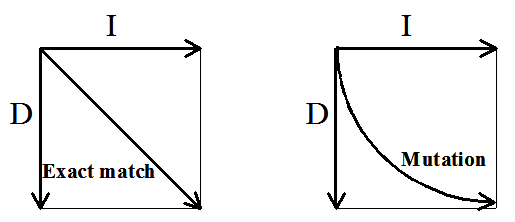Figure 3. Distinguishing between substitutions: an exact match is represented by a diagonal arrow (left panel) and a mutation is depicted by and arc (right panel).

Figure 4A depicts the alignment graph of S1 and S2 that utilizes straight diagonals for exact matches and arcs for mutations.  Figure 4B depicts the red and blue paths from Figure 2 on this more refined graph.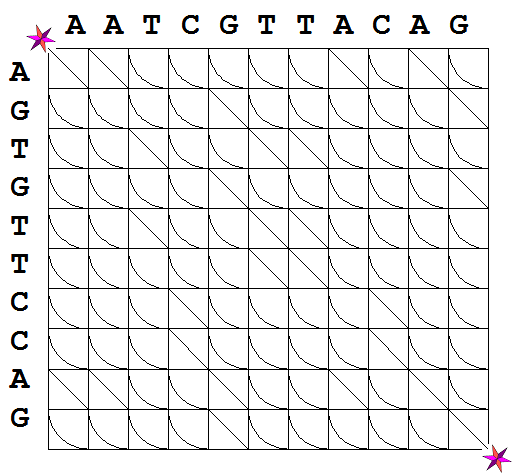Panlel A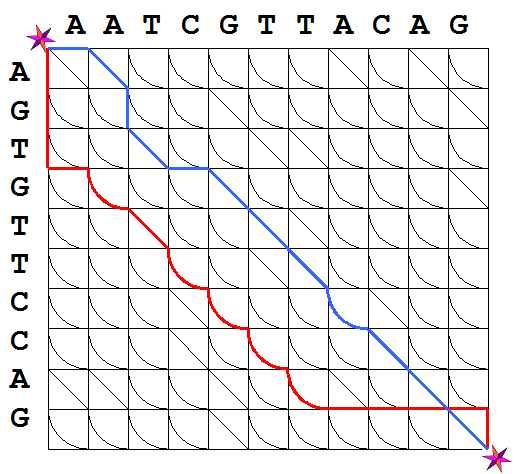Panel B Figure 4. Panel A: The weighted alignment graph for the sequences S1 and S2; Panel B: The weighted paths on the graph corresponding, respectively, to the red and blue paths from Figure 2.

The alignment graph with designated costs for each of its edges is the weighted alignment graph for the sequences S1 and S2.  The cost of a path is defined as the sum of the costs of all of its edges.

Example 2. The cost of the red path in Figure 4B is 9x + 5p. To see this, notice that the path corresponds to an alignment that contains 5 insertions (corresponding to five vertical edges), 4 deletions (corresponding to the total of horizontal edges in the path), 5 mutations and 1 exact match. Recall that that the cost of any insertion/deletion is x, the cost of a mutation is p, and the cost of an exact alignment is zero. Adding the individual weights for all edges in the path establishes the claim.

Exercise 5. Find the costs of the blue and black paths depicted in Figure 2A.

Clearly, the optimal alignment will correspond to a path of minimal cost. Thus,

 Finding an optimal alignment is the same as determining a path of minimal cost on the weighted alignment graph.

This problem may not seem too difficult at first glance, since the alignment graph has a finite number of edges. The following natural strategy, however, does not work in practice:

• Find all paths on the graph,
• Determine the cost of each path,
• Select the path of least cost.
The reason this idea does not work is that the number of paths in such a graph grows extremely rapidly with the increase of the sequences’ lengths, and the task quickly becomes computationally overwhelming. It can be shown that there are 224,143 different paths from the upper left to the lower right verteces on the alignment graph of two sequences of length n = 7 and m = 9. When, as is common in biology, we are attempting to align sequences that may be hundreds of bases long, making a complete list of all paths becomes intractable.

Fortunately, the mathematical problem of finding the paths of minimal cost on weighted graphs is a classical problem, and a number of computationally efficient solution strategies and algorithms are available. An important feature of these algorithms is that they do not need to compute the costs of all paths in a graph to find the shortest path. Dijkstra's algorithm is a well-known algorithm that works efficiently on any weighted directed graph. The Needelman-Wunsch algorithm is a special verion of this algorithm that can be used in our situation because alignment graphs have special properties. (The important properties here are that (1) the vertices can be laid out in a rectangular grid, and (2) all edges go down and/or to the right.) We will not discuss here the details of these algorithms as they can be found in many introductory books on discrete mathematics or algorithms and data structures. Instead, we have included an interactive applet that implements a shortest path algorithm and allows for changeing the costs x and p and observing how these changes affect the optimal alignment.

In closing, we emphasize that we have now described four equivalent ways of representing an alignment of two sequences S1 and S2:

1. as alignment strings, i.e., as tables of pairwise edits with hyphens in S1 standing for insertions and hyphens in S2 for deletions;
2. as edit strings, i.e., as sequences comprised of the letters I, D, and S;
3. as paths on the alignment graph; and
4. as paths on the weighted alignment graph.
The last representation is one for which computationally efficient mathematical solutions are known.